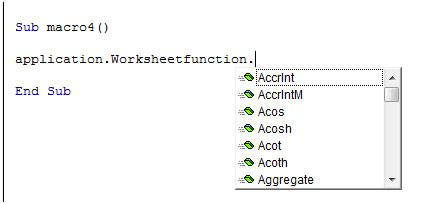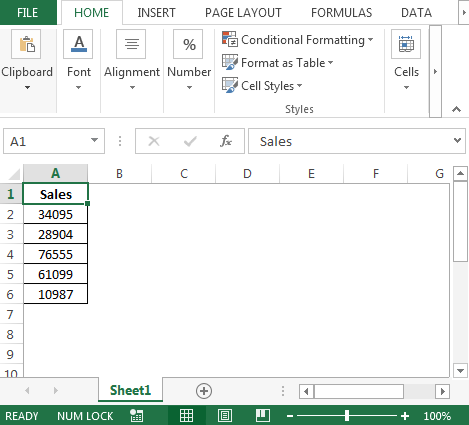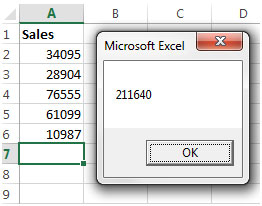# Worksheet functions in VBA macros using VBA in Microsoft Excel

In VBA code, if you have to refer to certain worksheet functions like Sum, Vlookup, etc, you can use them directly by using the Application object.  So we will use the functions as –

Application.WorksheetFunction.Sum or Application.WorksheetFunction.Vlookup where WorksheetFunction is the method of the Application object.

Considering we have this test macro, if we type

Application.WorksheetFunction.

We will get a popup showing the formulae refer below imageSo for example, if you want to sum the values of this range in column A using vba –Lets have a variable called SalesTotal which will save the sum in it.  To get total in SalesTotal we will use the following VBA code in standard module:

Sub macro4()
Dim SalesTotal As Long
SalesTotal = Application.WorksheetFunction.Sum(Range("A2:A6"))
MsgBox SalesTotal
End Sub

To copy the above code to your file,

• Press Alt + F11 on the keyboard.
• On the left hand side, you will see Microsoft Excel Objects.
• Right click and select Insert.
• Then click on Module.
• Copy the code to the code window on the right.

When we use this sample macro, we will get a message showing the value which is stored in Sales Total and can be used in further code lines in the macro.

The output we will get is -If you want the SalesTotal to be shown in cell A7, then you can change the code line from

Msgbox SalesTotal to Worksheets(“Sheet1”).Range(“A7”).Value = SalesTotal

Considering we also need the average sales for these figures in cell A9. We can use the code below

Sub macro5()
Dim SalesTotal As Long
With Worksheets("Sheet1")
SalesTotal = Application.WorksheetFunction.Sum(.Range("A2:A6"))
.Range("A7").Value = SalesTotal
.Range("A9").Value = Application.WorksheetFunction.Average(.Range("A2:A6"))
End With
End Sub

There are slight changes to this code as compared to the previous one.

• Instead of using a variable for the Average, I directly populated that value after calculation into cell A9.  Hence, you will see the line .Range(“A9”).Value = Application.WorksheetFunction.Average(.Range(“A2:A6”))
• The msgbox line has been removed.
• The value in the variable SalesTotal goes to cell A7.
• Ideally, you can repeat the same code line which has been used for the average, for the Sum code line by replacing

SalesTotal = Application.WorksheetFunction.Sum(.Range(“A2:A6”))

.Range(“A7”).Value = SalesTotal

With

.Range(“A7”).Value = Application.WorksheetFunction.Sum(.Range(“A2:A6”))

However, it has been retained so that you can understand the different ways of coding the same task. Similarly, we can use other functions while using Application.WorksheetFunction.  All those functions which we can use in the worksheet directly, we can use them here.

This makes it easier to use the functions in the code so we can calculate the formulae required without having to create a code for the formula.We would love to hear from you, do let us know how we can improve, complement or innovate our work and make it better for you. Write us at info@exceltip.com

## Users are saying about us...

1.I´ve tried to use some date functions, such as networkdays, date and year, on the vba enviroment but i had no success, do you have any tip for this kind of functions.

2."If you want to use functions from the Analysis Tool Pack addin in your own macros:
- Open the VBE (Alt+F11).
- Activate the project where you want to use the function(s).
- Select Tools, References... and check the option atpvbaen.xls.
- click the OK-button to close the References-dialog.

The macros in the workbook where you added the reference to the atpvbaen.xls library can now use the functions like this:

workdaycount = networkdays(Date, Date + 14)

Or like this to avoid conflict with other user defined functions with the same name:

workdaycount = [atpvbaen.xls].networkdays(Date, Date + 14)

The DATE() and YEAR() worksheet functions are not necessary in VBA where you use the built-in functions Date and Year:

Today = Date
Tomorrow = Date + 1
CurrentYear = Year(Date)
NextYear = Year(Date) + 1 "

3."when I use worksheet functions, arguments in functions are separeted by semicolon otherwise they dont work . for instance if I separate them with comma.
i.e.
OFFSET(Sheet1!\$E\$8,COUNT(Sheet1!\$E:\$E)-1,0) dosent work.
OFFSET(Sheet1!\$E\$8;COUNT(Sheet1!\$E:\$E)-1;0) works"

4."I think this is an OS setting - could be Excel in a version I am not familar with though.

What OS and version of Excel are you using? "

5.Hello, My name is Juan Macedo, I have some problems with Spanish Excel functions, that could be interesting for You:
I found these in Office different versions (last in Office 2013)
The Excel Address function is translated to DIRECCION, the Excel Offset function is translated to DESREF, well in Excell worksheet is possible to use the spanish function, but in VBA MACROS it is impossible to use any of both (English or Spanish versions), for some of the functions it is possible to use the english version when the spanish function is not available, but at least the two mentioned do not work.

Thanks

Juan Macedo
juan.mac2@gmail.com

6.That works for the explicit reference to worksheet cells using "A2:A6".

My question is how to use this function on an array within VBA, so that I can avoid writing to the workbook. Such as this:

DIM foo(3) as double
test(1)=10
test(2)=5
test(3)=1
Application.WorksheetFunction.Sum(test())
'which does not work, or
Application.WorksheetFunction.Sum(test(1:3))
'which does not work, etc

I cannot figure out the syntax to make this work. Thanks.

7.Sorry, corrected example:

DIM test(3) as double
test(1)=10
test(2)=5
test(3)=1
Application.WorksheetFunction.Sum(test())
‘which does not work, or
Application.WorksheetFunction.Sum(test(1:3))
‘which does not work, etc
Thanks.

Terms and Conditions of use

The applications/code on this site are distributed as is and without warranties or liability. In no event shall the owner of the copyrights, or the authors of the applications/code be liable for any loss of profit, any problems or any damage resulting from the use or evaluation of the applications/code.